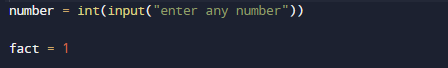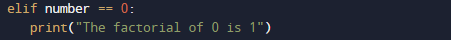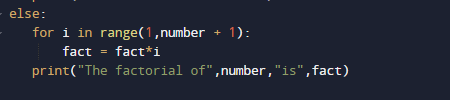# Python Program to Find the Factorial of a Number

In this tutorial you will learn about the Python Program to Find the Factorial of a Number and its application with practical example.

In this tutorial, we will learn to create a Python Program to Find the Factorial of a Number  using Python programming.

## Prerequisites

Before starting with this tutorial we assume that you are best aware of the following Python programming topics:

• Basic Input and Output
• Basic Python  programming.
• Python if-else statements.
• Python Loop.

## What is Factorial ?

Factorial of a given number ‘n’ is the product of all its positive integers numbers.

Example: The factorial of 5 is 120.
5! =  5 * 4 * 3 * 2 *1
5! = 120.

## Python Program to Find the Factorial of a Number

In this program we will find factorial of a given number . We would first declared and initialized the required variables. Next, we would prompt user to input a number .Later we will Find Factorial.

## OutputIn the above program, we have first declared and initialized a set variables required in the program.

• number = it will hold entered number.
• fact = it will hold a factorial of a number.
• i =  for iteration.

In this program, First we declare and initiate variables and later we will find factorial of a given number.
First of all we take a number as input from user and store the value in variable ‘number’. and fact  to one ( fact=1).And now we will check first that the given number is greater than 1 or not ( number< 0 ) if not we show message in below.Now we will check condition for number is equal to zero ( number ==0 ).As we know that if number is zero it’s factorial is one and after all his we finally find factorial of a given number.In this  program user is promoted to enter a positive integer as show in image above , the  for loop runs from ‘1 to number’. In every iteration , fact is multiplied with i ( fact=fact*i ) and after all iterations we will get the value of factorial of number.In this tutorial we have learn about the Python Program to Find the Factorial of a Number and its application with practical example. I hope you will like this tutorial.